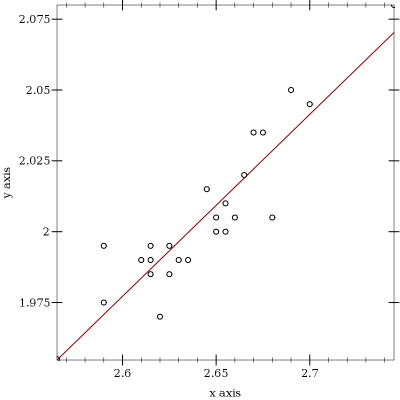Version: 5.2.1

## Least Squares: fitting a line to a sequence of 2d-points

Danny Yoo <dyoo@hashcollision.org>

 (require (planet dyoo/least-squares:1:=0))

This is a simple implementation of the least squares method for lines, described in a standard statistics textbook.

Sample usage:

> (require (planet dyoo/least-squares))
> (least-squares '((0 -0.2342) (1 1.0001) (2 1.82123) (3 3.1415926)))
 1.09485 -0.210096
 > (define my-linear-function (least-squares-function '((0 -0.2342) (1 1.0001) (2 1.82123) (3 3.1415926))))
> (my-linear-function 0)

-0.2100955200000003

> (my-linear-function 1)

0.8847552599999999

> (my-linear-function 2)

1.9796060400000002

> (my-linear-function 3)

3.0744568200000004

A slightly larger example:
 > (define data '(#(2.745 2.08) #(2.7 2.045) #(2.69 2.05) #(2.68 2.005) #(2.675 2.035) #(2.67 2.035) #(2.665 2.02) #(2.66 2.005) #(2.655 2.01) #(2.655 2.0) #(2.65 2.0) #(2.65 2.005) #(2.645 2.015) #(2.635 1.99) #(2.63 1.99) #(2.625 1.995) #(2.625 1.985) #(2.62 1.97) #(2.615 1.985) #(2.615 1.99) #(2.615 1.995) #(2.61 1.99) #(2.59 1.975) #(2.59 1.995) #(2.565 1.955)))
> (least-squares data)
 0.642098 0.307735
> (require plot)
 > (plot (list (points data) (function (least-squares-function data))))(least-squares data)
 [slope number] [intersect number]
data : (sequenceof (sequence number number))
Computes the slope and intersect for a line that best fits the points according to the method of least squares.

For example:
> (least-squares '((1 2) (3 4) (5 6)))
 1 1
> (least-squares '((2.718 3.1415926) (1.618 1.414213)))
 1.57035 -1.12661

 (least-squares-function data) → [f (number -> number)] data : (sequenceof (sequence number number))
Constructs a function that fits the given data. For example:
 > (define g (least-squares-function '((2.718 3.1415926) (1.618 1.414213))))
> g

(least-squares-function 1.5703450909090884 -1.1266053570909036)

> (g 0)

-1.1266053570909036

> (g 1)

0.44373973381818477

> (g 2)

2.014084824727273

> (g 3)

3.584429915636362

The function returned from least-squares-function is actually a structured value whose slope and intersect can be queried with least-squares-function-slope and least-squares-function-intersect.
 (least-squares-function-slope f) → number f : least-squares-function?
 (least-squares-function-intersect f) → number f : least-squares-function?
Example:
 > (define h (least-squares-function '((2.718 3.1415926) (1.618 1.414213))))
> (least-squares-function-slope h)

1.5703450909090884

> (least-squares-function-intersect h)

-1.1266053570909036

 (least-square-function? x) → boolean x : any
Returns true if x is a function produced by least-squares-function. Example:
> (least-squares-function? (lambda (x) x))

#f

> (define k (least-squares-function '((0 0) (1 1))))
> (least-squares-function? k)

#t